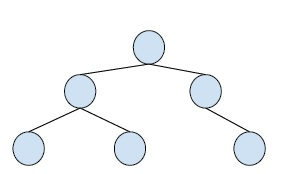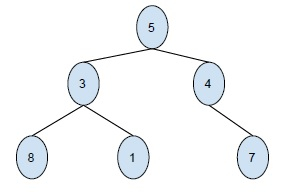# Print Binary Tree in 2-Dimensions in C++

C++Server Side ProgrammingProgramming

#### C in Depth: The Complete C Programming Guide for Beginners

45 Lectures 4.5 hours

#### Practical C++: Learn C++ Basics Step by Step

Most Popular

50 Lectures 4.5 hours

#### Master C and Embedded C Programming- Learn as you go

66 Lectures 5.5 hours

In this problem, we are given a binary tree and we have to print it two dimensional plane.

Binary Tree is a special tree whose every node has at max two child nodes. So, every node is either a leaf node or has one or two child nodes.

Example,Let’s take an example to understand the topic better −Output -

      7
4
5
1
3
8

Now as we have seen in the example, the nodes of the tree are printed in a 2-D output screen horizontally.

Here, we have flipped the tree by 90o.

Let’s see what the new horizontal tree is made up of,

• The tree data structure is stored in a horizontal way which includes

• The root at the 1st position in horizontal view n lines below the starting line. i.e. root will be at the start of the nth line.

• The new levels of the tree are in lines n+i and n-i. And at i tab spaces away from the start of the line.

• And the rightmost leaf node of the tree is printed in the first line. Whereas the leftmost node of the tree is printed at the last line.

## Example

Let’s create a program based on this logic −

Live Demo

#include<bits/stdc++.h>
#include<iostream>
using namespace std;
#define COUNT 10
class Node{
public:
int data;
Node* left, *right;
Node(int data){
this->data = data;
this->left = NULL;
this->right = NULL;
}
};
void printTree(Node *root, int space){
if (root == NULL)
return;
space += COUNT;
printTree(root->right, space);
for (int i = COUNT; i < space; i++)
cout<<"\t";
cout<<root->data<<"\n";
printTree(root->left, space);
}
int main(){
Node *root = new Node(43);
root->left = new Node(25);
root->right = new Node(67);
root->left->left = new Node(14);
root->left->right = new Node(51);
root->right->left = new Node(26);
root->right->right = new Node(97);
root->left->left->left = new Node(81);
root->left->left->right = new Node(49);
root->left->right->left = new Node(07);
root->left->right->right = new Node(31);
root->right->left->left = new Node(29);
root->right->left->right = new Node(13);
root->right->right->left = new Node(59);
root->right->right->right = new Node(16);
printTree(root, 0);
return 0;
}

## Output

         16
97
59
67
13
26
29
43
31
51
7
25
49
14
81